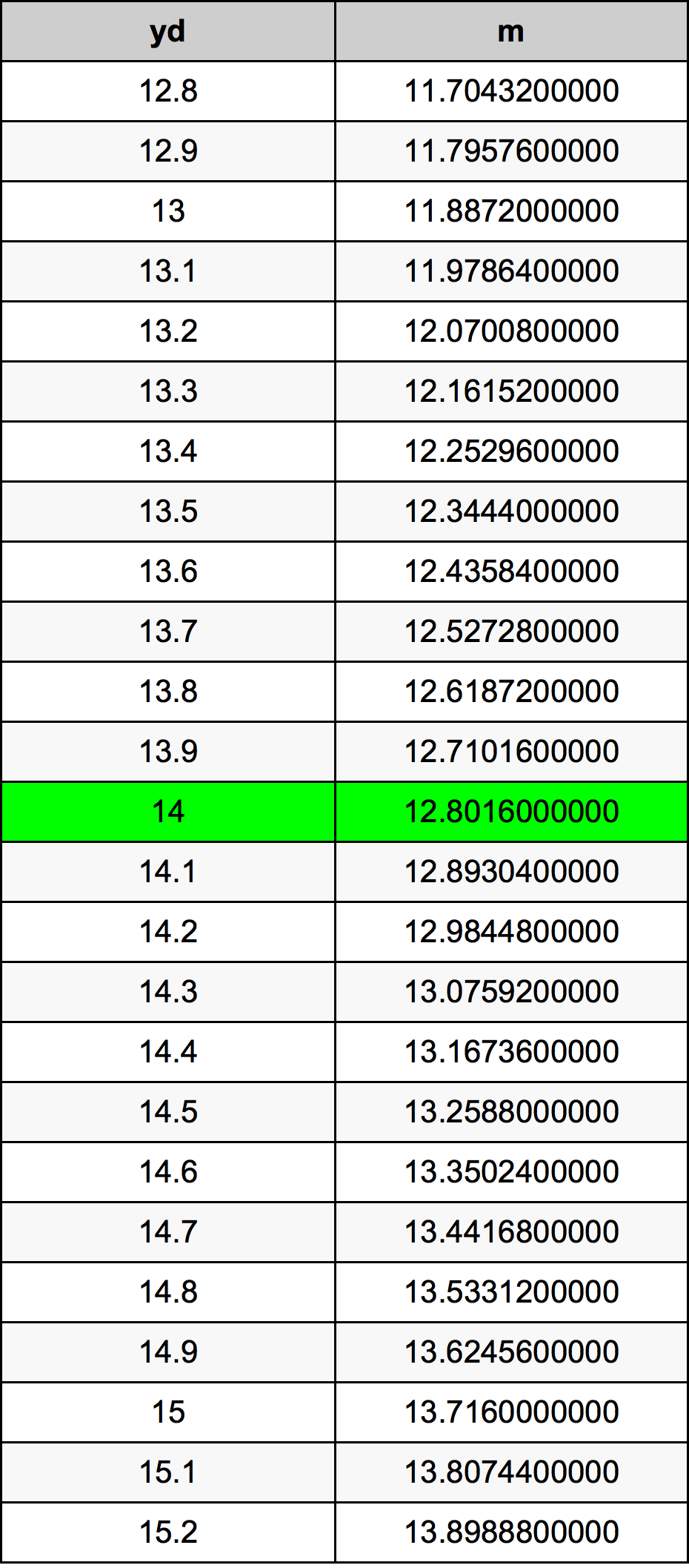Yards To Meters

# 14 yd to m14 Yards to Meters

yd
=
m

## How to convert 14 yards to meters?

 14 yd * 0.9144 m = 12.8016 m 1 yd
A common question is How many yard in 14 meter? And the answer is 15.3105861767 yd in 14 m. Likewise the question how many meter in 14 yard has the answer of 12.8016 m in 14 yd.

## How much are 14 yards in meters?

14 yards equal 12.8016 meters (14yd = 12.8016m). Converting 14 yd to m is easy. Simply use our calculator above, or apply the formula to change the length 14 yd to m.

## Convert 14 yd to common lengths

UnitLengths
Nanometer12801600000.0 nm
Micrometer12801600.0 µm
Millimeter12801.6 mm
Centimeter1280.16 cm
Inch504.0 in
Foot42.0 ft
Yard14.0 yd
Meter12.8016 m
Kilometer0.0128016 km
Mile0.0079545455 mi
Nautical mile0.006912311 nmi

## What is 14 yards in m?

To convert 14 yd to m multiply the length in yards by 0.9144. The 14 yd in m formula is [m] = 14 * 0.9144. Thus, for 14 yards in meter we get 12.8016 m.

## 14 Yard Conversion Table## Alternative spelling

14 yd to m, 14 yd in m, 14 Yard to m, 14 Yard in m, 14 Yard to Meter, 14 Yard in Meter, 14 Yards to Meters, 14 Yards in Meters, 14 Yards to m, 14 Yards in m, 14 Yards to Meter, 14 Yards in Meter, 14 yd to Meters, 14 yd in Meters